Date: Sat, 1 Feb 1997 11:02:48 +0800 (HKT)

Hello ,
I am Ronald.

Question:
Determine all ordered pairs (m,n) of positive integers such that ( n^3+1)/(mn-1)is an integer.

Thank you very much!!!!!!!!

From ,
Ronald

Hi Ronald

First note that gcd(n,mn-1) = gcd(m,mn-1) = 1 and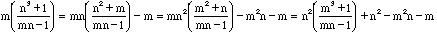. Therefore if one of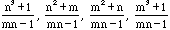is an integer they all are.

Now suppose that (*)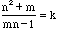is an integer. Notice thatif and only if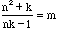, so we can interchange m and k if necessary to get 0<m<=k. Since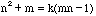we have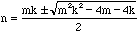and so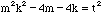for some integer t >= 0. Thus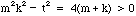which implies that mk > t and that mk and t are both even or both odd. Therefore mk - t >= 2.

Now 2(mk + t) <= (mk-t)(mk+t) <= 4(m + k) <= 8k which implies mk <= 4k and thus m=1, 2, 3 or 4.

For these choices of m the expression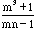has values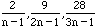and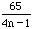respectively. For these to be integers we have n=2 or 3 in the first case, 1,2, or 5 in the second case, 1 or 5 in the third case and the fourth case in never an integer. Thus from expression (*) the admissible values of m, n and k are

 m 1 1 2 2 2 3 3 n 2 3 1 2 5 1 5 k 5 5 3 2 3 2 2
Therefore there are 9 solutions to the original problem, (m,n) =(1,2), (2,1), (1,3), (3,1), (2,5), (5,2), (3,5), ( 5,3) and (2,2).

Richard

Go to Math Central